Practice Sine and Cosine Graphs Terms of Use    Contact Person: Donna Roberts1.
Determine an equation for this graph: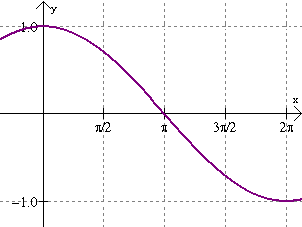Choose: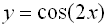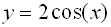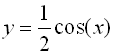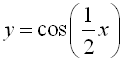2.
Determine an equation for this graph: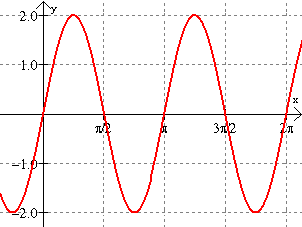Choose: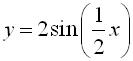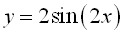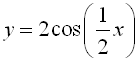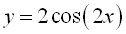3.
Determine an equation for this graph: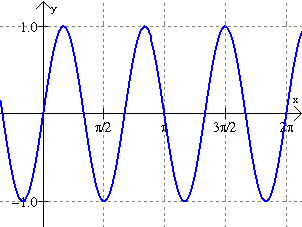Choose: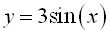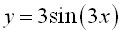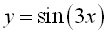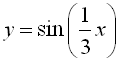4.
Determine an equation for this graph: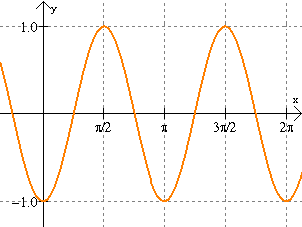Choose: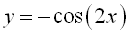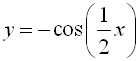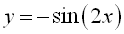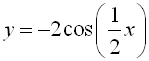5. On the same set of axes from 0 to 2π, graph: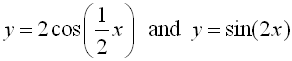6 On an axes from 0 to 4π, graph: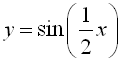. Where on the graph does the graph reach its maximum value(s)?7 On the same set of axes from 0 to 2π, graph: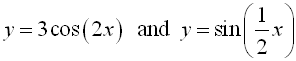How many points satisfy the equation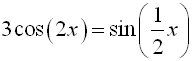?8 On the axes from 0 to 2π, graph: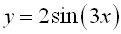. State the amplitude, frequency and period of this graph.9 On the same set of  axes,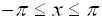, graph: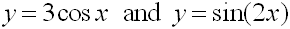. Find all values for which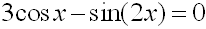10 A small toy attached to the end of a slinky (or spring) bobs up and down according to an equation of the form d = a cos(bt).  The motion of the toy starts at its highest position of 5 inches above its rest point, bounces down to its lowest position of 5 inches below its rest point, and then bounces back to its highest position in a total of 4 seconds.  Write an equation that represents this motion. Graph your equation from t = 0 seconds to t = 12 seconds.